# Discounting cash flows

Discounting cash flows is another very important aspect in finance and is actually not very different from interest rate compounding. Discounting is used when people want to determine the present value of a future amount of capital.

## Basics on discounting cash flows

In the following formula we will start off by demonstrating an interest rate calculation for 1 year. B represents the starting capital, r represents the interest rate on a yearly basis and E equals the ending value of your capital. Instead of trying to determine the ending capital, our objective is now to determine the starting capital B based on r and E. The solution is simple. We can just rearrange the basic interest equation to the following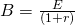If the interest rate r equals 10% and you know that in 1 year E will equal 1100, we perform the following calculation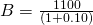and find that B equals 1000.

## Multiyear discounting

Discounting over multiple years is done in a similar way. To this end we just add a power t to the previous formula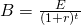This t represent represents time and is expressed in years. If the interest rate r equals 10% per annum (i.e. per year) for a 2 year investment horizon, and the ending value E equals 1210 in 2 years from now, then by discounting this 1210, we find today it is worth a 1000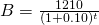## Multi-period discounting

Determining the value of an asset generating cash flows over multiple years in the future is just as simple. We extend the previous formula by taking the sum of 2 discounted cash flows. The first cash flow is discounted 1 year using the annual interest rate on a 1 year investment horizon. The second cash flow is discounted using the annual interest rate on a 2 year investment horizon.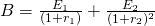Specifically suppose you get a value of 2000, spread equally over two years. Additionally, both the 1-year and 2-year investment horizon interest rates are 10% per year.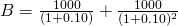Solving this equation will  of B is then equal to 1735.54. This result can easily be further extended to even longer horizons. Also note that it is not required that the cash flows or the interest rates are the same across all years.

## Summary

Discounting cash flows is another essential part of any investor’s skill set. It is used to determine the value of an asset today based on future cash flows and current interest rates.

### Discounting cash flows

Need to have more insights? Download our free excel file: discounting cash flows.